# Symmetric

Symmetric – Identical parts facing each other. The simplest type is a mirror.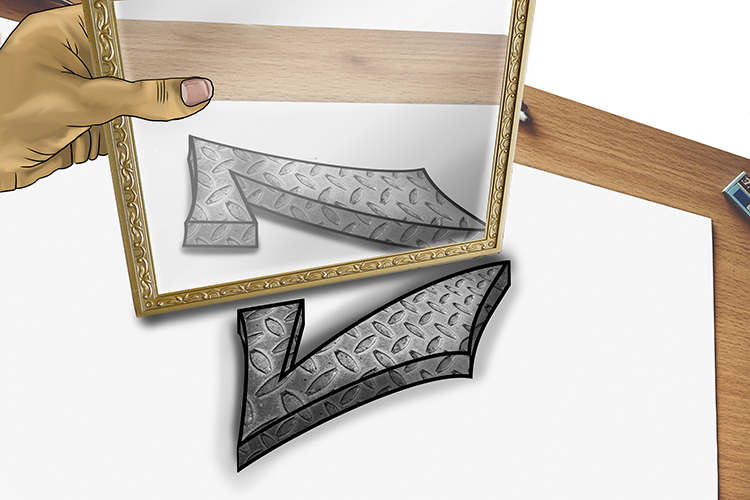See metal tick (symmetric) with a mirror. (Mirrors always provide a symmetrical view in reflection.)

Example 1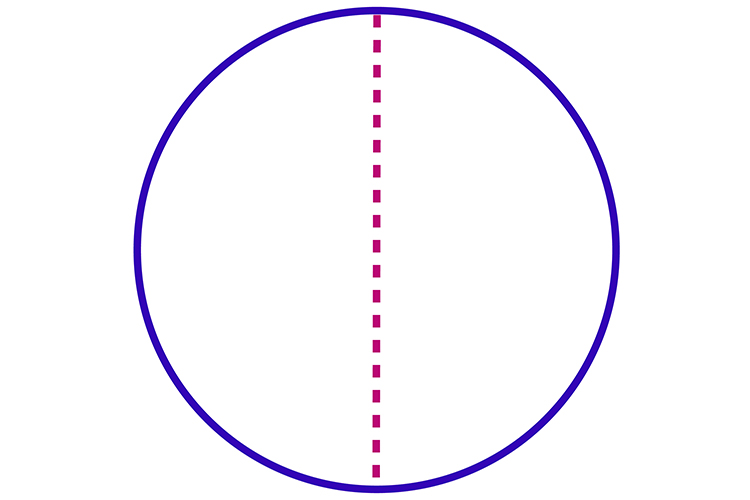A circle is symmetrical through any line that divides it perfectly in half.

Example 2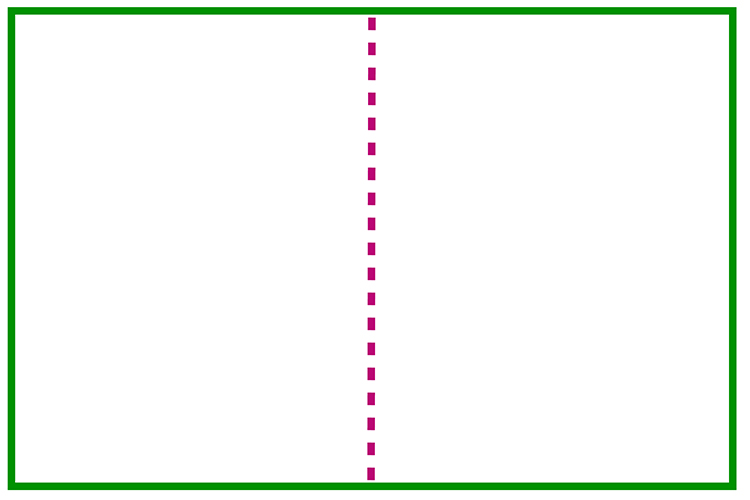A rectangle is symmetrical about a vertical line through the centre, and a horizontal line through the centre.

Example 3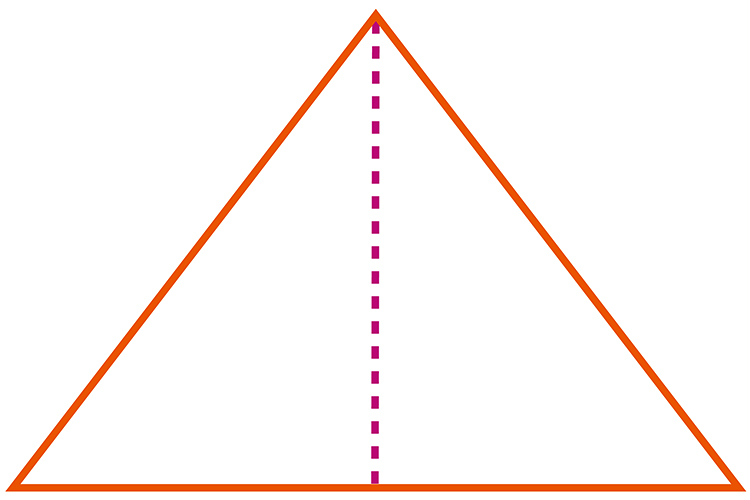This triangle is symmetrical about the vertical line passing through its centre.

Example 4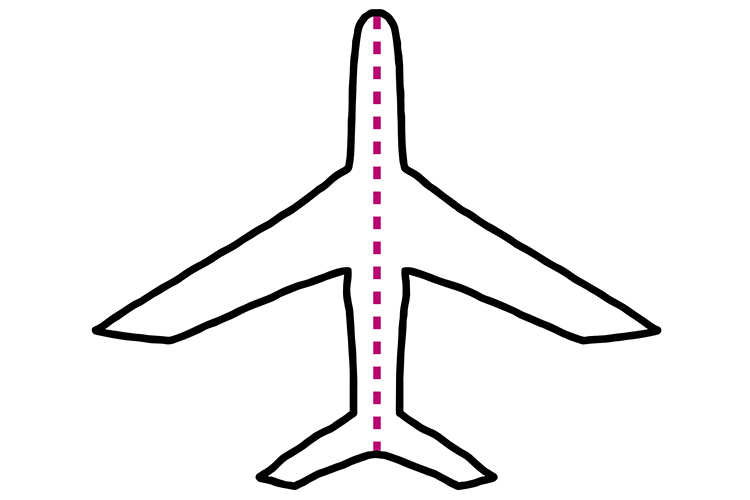This plane shape is symmetrical about the red line that passes through it.

Example 5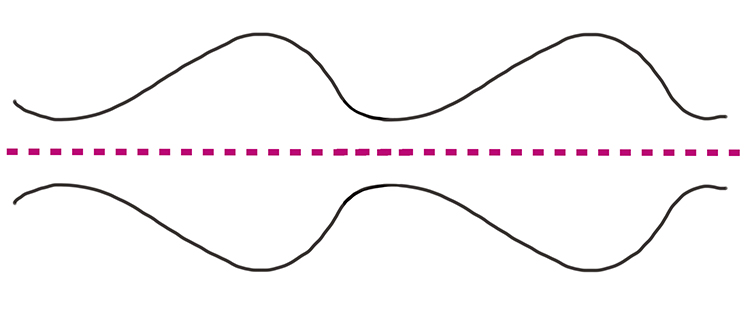Lines such as the ones above or those on a graph may also be symmetrical.